# Back to Riemann, Part 15

There’s just one final thing I want to address in this series, and that’s how to implement the gamma function.

The Wiki article starts out with the following definition:
“Find a smooth curve that connects the points (x, y) given by y = (x – 1)! at the positive integer values for x.”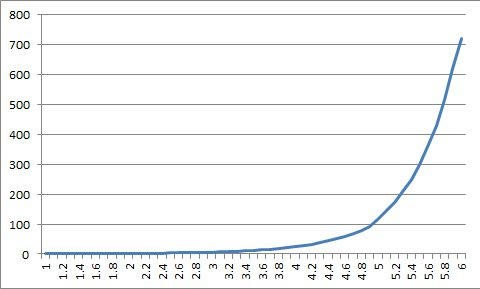This is related to the factorial curve that I presented in part 9. While the answer can be found through integration and the use of limits, one of the more commonly used approaches is through the gamma function.

This formula isn’t very easy to use, so the next step is to look at the Lanczos approximation, published by Cornelius Lanczos in 1964.

where Ag(z) is:

then, g is a constant we choose such that the real part of z > 1/2. The pn coefficients can then be pre-calculated for a given g, using the formula in the wiki article. I’m not going to keep reproducing all these equations here, because then I’d have no room left for my part of the writing.

But, if you pre-calculate the constants, Ag(z) becomes:

Which leads me to the next part, which is the Python code example for calculating gamma. I’ve never used Python, and there are a couple parts that I had trouble understanding with just a cursory glance at the code. But, it seems that Python has a full implementation for complex number handling, which is nice. The thing is, I prefer VBScript because it’s (normally) easy to use, it comes with Windows, it’s free, the implementation doesn’t need to be downloaded from somewhere, I can run it directly from the command line, and I know how to tie it to Excel for drawing graphs. The downside is that VBScript has almost nothing in the way of math support, can’t handle complex numbers, and doesn’t support making classes.

In fact, VBScript is almost useless for calculating gamma, but I’m looking at this as a recreational math project, which could be an interesting learning experience. Plus, I’m almost done with the code now, so there’s little reason to quit at this stage. (Ha ha.)

First things first. We need a way to represent complex numbers. I’m going to use a simple 2-element array, and my own function naming conventions.

‘ Create two constants to act as array index constants,
‘ re for the real component, im for the imaginary one.
re = 0
im = 1

‘ Create a 2D array to hold the complex number: c(re) = a, c(im) = bi
Function complex(a, b)
dim cplx(2)

cplx(re) = a
cplx(im) = b
complex = cplx
end Function

‘ Return the real component of the complex number.
Function complexReal(c)
complexReal = c(re)
end Function

‘ Return the imaginary component of the complex number.
Function complexImg(c)
complexImg = c(im)
end Function

‘ Return the magnitude of the complex number (sqrt(a^2 + b^2))
Function complexMag(c)
complexMag = (c(re)^2 + c(im)^2)^0.5
end Function

Then, I want a few simple functions for handling multiplication and addition of complex numbers. Initially, I planned on having separate functions for pure complex numbers, and for mixed scalars with complex numbers. And, I was going to recast scalars as complex numbers in-line when needed. But that started getting really clunky and hard to read. So I figured I might as well put a test for whether a variable was an array within the function, and if it wasn’t, then I’d re-cast it. In my version of VBScript, vartype(array) returns 8204.

‘ Test if a variable is an array, and if not, return an array
Function makeComplex(mCc)
dim cplx(2)

if(vartype(mCc) <> 8204) then
cplx(re) = mCc
cplx(im) = 0
makeComplex = cplx
else
makeComplex = mCc
end if
end Function

‘ a1 + a2 + i*(b1 + b2)
Function complexSum(c1, c2)
dim cplx(2)

tc1 = makeComplex(c1)
tc2 = makeComplex(c2)

cplx(re) = tc1(re) + tc2(re)
cplx(im) = tc1(im) + tc2(im)
complexSum = cplx
end Function

‘ (a1 + b1*i) * (a2 + b2*i)
Function complexProd(c1, c2)
dim cplx(2)

tc1 = makeComplex(c1)
tc2 = makeComplex(c2)

cplx(re) = tc1(re) * tc2(re) – tc1(im) * tc2(im)
cplx(im) = tc1(re) * tc2(im) + tc1(im) * tc2(re)
complexProd = cplx
end Function

At some point, we’re going to need to take the sine of a complex number. While VBScript supports sin(), cos(), exp() (which is actually e^x) and log() (actually the natural log = ln()), they handle real numbers only. Fortunately, this is where the Internet steps in to save us. PlanetMath.org shows the first part.

sin(x+i*y) = sin(x)*cosh(y) + i*cos(x)*sinh(y)
cos(x+i*y) = cos(x)*cosh(y) – i*sin(x)*sinh(y)

But, VBScript doesn’t support hyperbolic sines and cosines. However, vbsedit.com has a page of Derived Math Functions, which is EXACTLY what I need here.

sinh(X) = (Exp(X) – Exp(-X)) / 2
cosh(X) = (Exp(X) + Exp(-X)) / 2

‘ sinh(a) = (e^a – e^(-a)) / 2
Function sinh(sa)
sinh = (exp(sa) – exp(-sa))/2
end Function

‘ cosh(a) = (e^a + e^(-a)) / 2
Function cosh(sa)
cosh = (exp(sa) + exp(-sa))/2
end Function

‘ sin(a+bi) = sin(a)*cosh(b) + i*cos(a)*sinh(b)
Function complexSin(c)
dim cplx(2)

cplx(re) = sin(c(re)) * cosh(c(im))
cplx(im) = cos(c(re)) * sinh(c(im))
complexSin = cplx
end Function

‘ cos(a+bi) = cos(a)*cosh(b) – i*sin(a)*sinh(b)
Function complexCos(c)
dim cplx(2)

cplx(re) =   cos(c(re)) * cosh(c(im))
cplx(im) = – sin(c(re)) * sinh(c(im))
complexCos = cplx
end Function

I’ll end here, because I’ll be getting into how Python handles for-loops on arrays, and there’s one bit I’m having trouble understanding, so I need to figure that out first.

# Back to Riemann, Part 14

Finally, the culmination of all my messing around – some animations.

# Back to Riemann, Part 13

Ok, so I’m now ready to sit down and write up an Excel spreadsheet to graph the first few zeta zeroes. As I’m preparing for this, I noticed that I made a mistake in part 8 of the Riemann posts, a couple hours after I uploaded it to wordpress, so I had to go back and fix that. I’m also using part 8 for getting the math I need for the spreadsheet.

First, the zeta function is:
zeta(x) = 1 + 1/2^x + 1/3^x + 1/4^x + 1/5^x…

1/2^x translates to: (1/(2^a)) * (cos(b * ln(2)) – i*sin(b * ln(2)))

The generalized form is:
1/n^x = (1/(n^a)) * (cos(b * ln(n)) – i*sin(b * ln(n)))

Second, the eta function is:
eta(x) = 1 – 1/2^x + 1/3^x – 1/4^x + 1/5^x – 1/6^x…

Writing the generalized translated form of the eta function just means having alternating minus signs for the even terms.

Where things got trickier than I was expecting was with the conversion factor to go from the eta(x) value to the zeta(x) value.

zeta(x) = eta(x) / (1 – 2^(1-x))

Remembering that
2^x = 2^(a + bi) = (2^a) * (2^bi) = (2^a) * e^(bi * ln(2))
e^bi = cos(b) + i*sin(b)
(2^a) * e^(bi * ln(2)) = (2^a) * (cos(b * ln(2)) + i*sin(b * ln(2)))

This gives:
1/(1 – 2^(1-x)) = 1/(1 – ((2^(1-a)) * (cos(b * ln(2)) + i*sin(b * ln(2)))))

Or, since a = 1/2,
1/(1 – sqrt(2) * cos(b * ln(2)) + i * sqrt(2) *sin(b * ln(2)))

Simplified:
1/(j + ik)

And, yeah, I didn’t remember how to take the reciprocal of a complex number, and this doesn’t seem to have been covered in the der Veen and de Craats book. Fortunately, this is one of the rarer cases where I could find the answer quickly on the net.

Step 1, find the conjugate of the complex number (i.e., j – ik).
Step 2, multiply the numerator and denominator by the conjugate.
Step 3, simplify.

If j = 1 – sqrt(2) * cos(b * ln(2))
and k = sqrt(2) * sin(b * ln(2))

(j – ik)/(j + ik)(j – ik)
(j – ik)/(j^2 + ijk – ijk + k^2)
(j – ik)/(j^2 + k^2)

Writing all this out here in the blog would be too messy. But, doing this in Excel isn’t that bad because I can treat “j” and “k” as cell names.

Getting the zeta function results is even messier, but in simplified form:
If eta(x) = m + in

zeta(x) = (j*(m+n) – ik*(m+n)) / (j^2 + k^2)
Believe me, it makes more sense when you put everything into Excel and refer to the cell names this way.

This then gives me two choices as to how to proceed next. First is to just do a simple spreadsheet for one zero, and write a little VBScript to update the spreadsheet to make an animated dot running around the graph. Second is to copy the first set of columns multiple times to draw a more detailed line segment showing the zeta going to zero.

The second approach has the advantage of overcoming some of the rounding errors introduced by Excel when adding up the zeta function terms. The results of the Excel sheet aren’t going to be all that accurate, so it’s not going to go to |zeta(x)| = 0, it’s just going to get close and then move away again. But that’s ok.

So, which approach did I pick?
#2

Without the VBscript animation part, this is what I’ve got for the first zero.This graph shows the real and imaginary components of zeta(x) for a=0.5 and b approaching the first zero at 14.134Here we’ve got the magnitude of zeta(x), and it’s pretty obvious it’s not going to zero. I blame rounding errors.And then, the zeta(x) function from a + ib = 0.5 + i*14.0 to 0.5 + i*14.5 in 0.05 steps on the complex plane. This is the line segment I’d animate with a VBScript driving the Excel graphs. If my math were perfect, the segment would be crossing the axes at 0,0. As it is, I have the eta(x) calculated out to 300 terms. Not sure if it’s worth the effort of going to 1,000 terms or not. Depends on how bored I get, I guess.

All that’s left now is to write the VBScript (I can modify the one I wrote for the first Riemann prose blog series), and to decide if I want to figure out a VBScript version of the Gamma approximation.

# Back to Riemann, Part 12

One of the issues I hadn’t covered in much detail in the last post was what happens if Riemann’s hypothesis fails somewhere. That is, what if one of the zeta zeros is not on the critical line x = 1/2.

Remember that in the explicit formula for the prime counting function, we’re summing up the effects of ALL of the zeros for a specific value of x (x being the range of counting numbers that contains the prime numbers we want). To make that easier to do, we pair up each zero with its mirror version: u = v + wi with mirror(u) = v – wi.

The equation for that zero-pair then becomes:

-(2/|u|) * (x^v) * cos(w*ln(x) – a)

If the zero lies on the critical line, we get:
-(2/sqrt((1/2)^2 + w^2) * sqrt(x) * cos(w * ln(x) – atan(w))

If it doesn’t, then we end up with two pairs of zeros:
v + wi, v – wi, 1 – v + wi and 1 – v – wi

And we get two equations:

-(2/sqrt((v)^2 + w^2) * x^v * cos(w * ln(x) – atan(w/v))
-(2/sqrt((1-v)^2 + w^2) * x^(1-v) * cos(w * ln(x) – atan(w/(1-v)))

Right away, it should be obvious that if v is really close to 0.5, that we’re going to have two results that are very similar, in effect doubling the impact of this zero on the total for the psi(x) summation. And, although size of w for the zero is going to be much larger than v is (say we use the 410th zero: 693.1769701, and v is 0.51), meaning that the variation in v won’t affect the absolute value of v + wi very much, the variations will become much more noticeable in the x^v and x^(1-v) parts as we calculate psi(x) for larger values of x (i.e. -> x = 100,000^(0.1) or 100,000^(0.9)).

Then, what would that look like? Well, first, it depends on how far from v = 1/2 the zeros are. We know that there are no zeros for v < 0 or v > 0, and it turns out that there are no zeros directly on the boundaries v = 0 or v = 1. In fact, the zeros are going to be closer to v = 1/2, anyway. But let’s assume that the rogue zero is somewhere between 0.1 and 0.9. If we have one zero at 0.9 + w, then we’ll get matching zeros at 0.9 – w, and 0.1 + w and 0.1 + w.

Now, let’s arbitrarily pick one of the zeros for w, say the 13th one, at 59.347044003.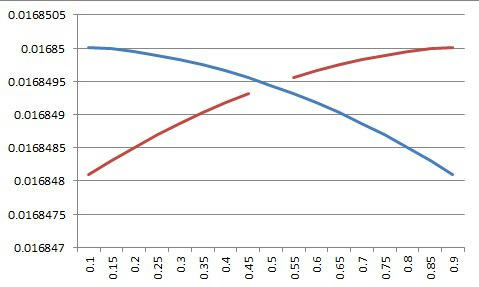Then, the plots for 2/sqrt((v)^2 + w^2) and 2/sqrt((1-v)^2 + w^2), with v running from 0.1 to 0.9 are above.
This is pretty flat.
If we add them together, remembering that we’re not counting v = 0.5 twice…They are flat, except that, as mentioned before, having 4 zeros instead of 2 is doubling the magnitude of these pairs.

Now, the place where we really get a lot more contribution from these zeros is in the parts x^v and x^(1-v). If v is 0.9 and 1 – v is 0.1, then if x is 1000 and v is 0.9, (2/(|u|)) * x^v = 8.4, compared with 0.533 when v=1/2.The reason for using x=1000 is simply that the contribution for (2/(|u|)) * x^0.5 is so small if x is small. Besides, looking at psi(1000) starts getting us up into somewhat larger values of x, and the entire point is what happens when x tends towards infinity.

Adding the results from the above graph together, we get:where the v-notch represents the smallest value for the contribution for v = 0.5 and w = 59.347044003. If the zeros here are off the critical line, they really throw off the results of the psi(x) approximation.(Correct approximation for psi(100.)

Compare the correct graph above, for psi(100), with the 11th zero set so that v=0.9 and 1-v=0.1, below.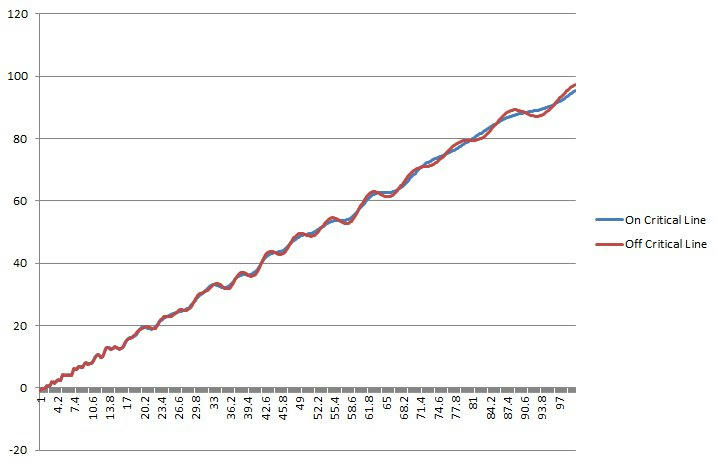(Summations of the first 11 zeros, comparing the correct values to v=0.1/v=0.9 for the 11th zero.)

der Veen and de Craats talk about the “music of the primes.” We saw this a little bit in the last blog entry. The cosine component for each non-trivial zero of the explicit formula creates a growing, slowing cosine result as x gets bigger. These ringing bell waveshapes get added together for ALL zeros, spanning the entire range of x, in the psi(x) equation. This is why the Riemann hypothesis is so important. If even one zero is off the critical line, you get the 2 pairs of zeros adding together instead of the one pair, throwing off the step-shape of the approximation. And that invalidates the prime number theorem.

The way der Veen and de Craats put it, each non-trivial zero, in the explicit formula, acts like the next octave up on the “musical scale”. These are smooth, tonal increments from one zero to the next. If any of the non-trivial zeros is not on the critical line, the amplitude of that doubled-up zero pair becomes an ugly discordant spike.

I’m showing the first 10 zero pairs from the explicit formula to show what I mean. I introduced the first and tenth zeros in the last blog entry. Adding them all together gives the approximation for psi(100) in the above graph.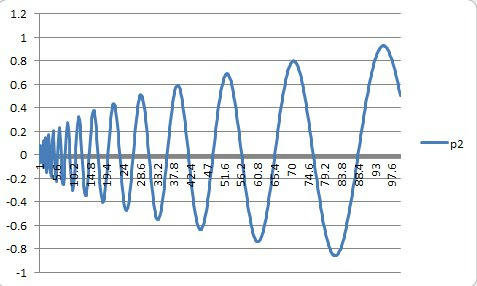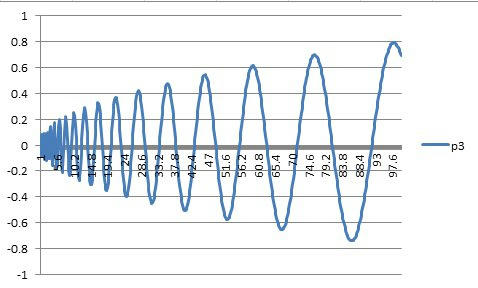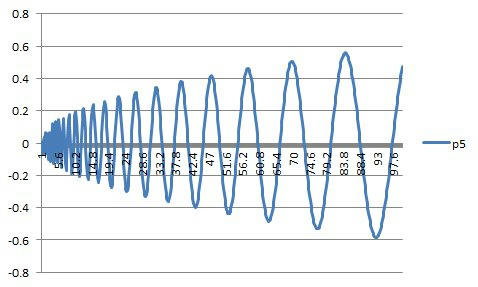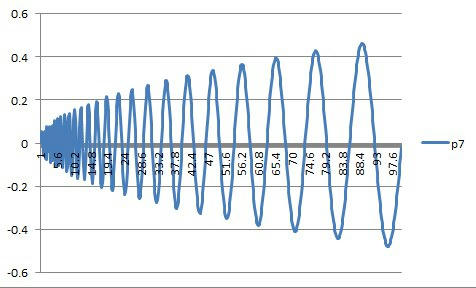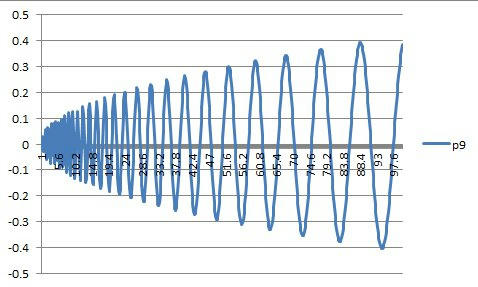Also, I just want to show the magnitude contributions for the first ten zeroes, as x goes to 6,250. The relative spacing between one zero and the next remains constant, independent of x.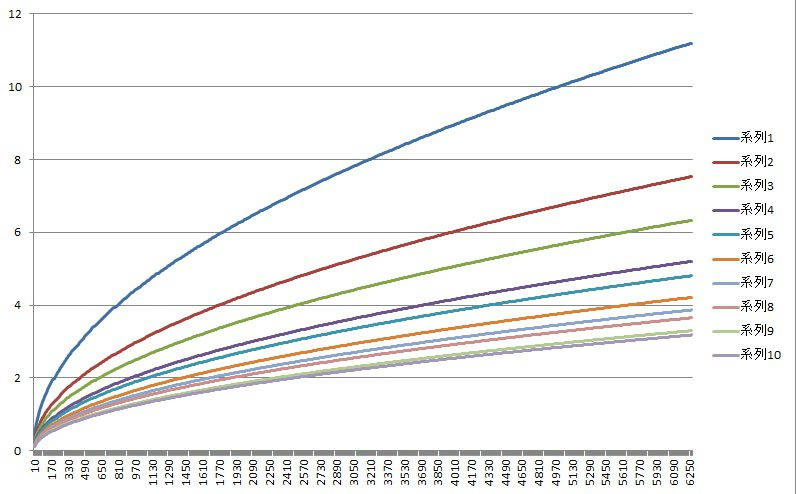But it’s not a linear, smooth or consistent spacing.(Left: Relative spacing of the contributions of the first 10 zeros for the explicit formula. Right: For comparison’s sake, the relative spacing for the first 10 prime numbers. Note that spacing of the zeros doesn’t reflect the spacing of the primes themselves.)

Anyway, the objective for this blog entry was just to consider the impact of having one of the non-trivial zeros not being on the critical line. The answer is that it really depends on how far off from the line the two matching zero pairs (v and 1 – v) are. If they’re very close to 0.5, then they just double the magnitude of the -(2/|u|) * (x^v) * cos(w*ln(x) – a) contribution for that zero.

If they’re farther apart, such as being at v = 0.9 and 1 – v = 0.1, then the results can be up to 8 times greater. It all depends on how big x is where you’re looking.

Having a proof showing that the Riemann hypothesis is true, or even that it is false, would remove the uncertainty from the question. After 158 years, we’re still waiting for someone to figure out what that proof is.